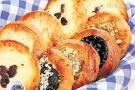# Dolores

Dolores has a number of cookies and she does not want to tell the exact number. Instead, she gives a riddle. First, multiply the number by 2. Then add 2000 to the result. After dividing the answer by 2, the result is 1036. How many cookies does Dolores have?

n =  36

### Step-by-step explanation:Did you find an error or inaccuracy? Feel free to write us. Thank you!

Tips for related online calculators
Do you have a linear equation or system of equations and looking for its solution? Or do you have a quadratic equation?